## Cost-Volume-Profit Analysis and Break-even point

Today we will take a look at Cost-Volume-Profit (CVP) analysis and the Break-even point (BEP) in sales.

# Cost-Volume-Profit Analysis

The Cost-Volume-Profit  (CVP) analysis is a method of cost accounting. It looks at the impact of changes in production costs and sales on operating profits. Performing the CVP, we calculate the Break-even point for various sales volume and cost structure scenarios, to help management with the short-term decision-making process. As it focuses mainly on the Break-even point, it is commonly referred to as Break-even Analysis.

When performing a CVP analysis, we need to consider the following inherent assumptions:

• Selling price is constant for varying quantities of sold units;
• Fixed Costs are consistent at the specified production levels;
• Variable Costs per unit do not vary between different numbers of produced units;
• All manufactured items are sold in the same period;
• Costs are classified as Fixed or Variable; no semi-fixed costs can exist in the analysis;
• All costs are only affected by changes in the activity.

The CVP formula shows us the sales volume needed to cover costs and break even. The recipe for the break-even sales is:We know where Fixed Costs come from, now let us look at the contribution margin.

## Contribution margin

To calculate the CVP equation, we first take a look at the Contribution Margin.The Contribution Margin (CM) is a basic calculation in CVP analysis. It represents the profit the company has made, to cover Fixed Costs. We can also calculate it on a per-unit basis. Another calculation is the Contribution Margin ratio: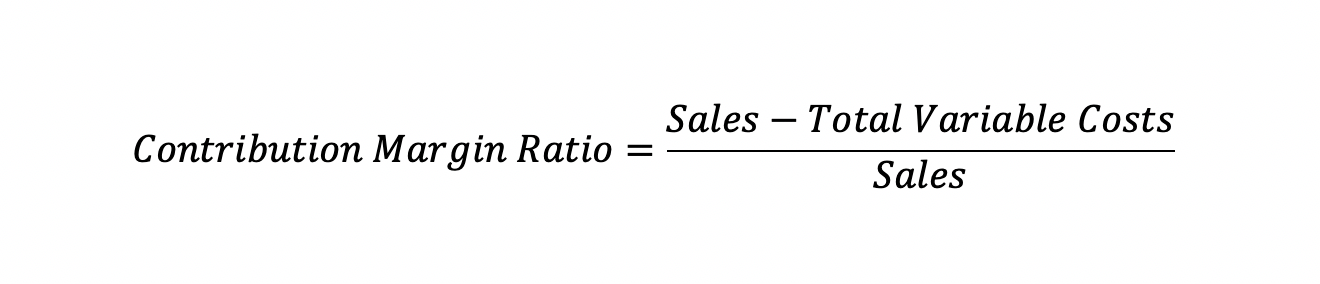We can conclude that whenever the Contribution Margin is more than the Fixed Costs, the business is profitable.

## Break-even point

The volume of production and sales where all relevant costs are covered by the revenue is called the break-even point (BEP). This is where the business breaks even and can start generating income. The BEP represents the level of sales where net income = zero, the point where Sales = Total Variable Costs + Total Fixed Costs, and Contribution Margin = Total Fixed Costs.

To calculate the break-even amount, we divide the Total Fixed Costs over the Contribution Margin ratio:Another way to calculate the break-even amount is as follows: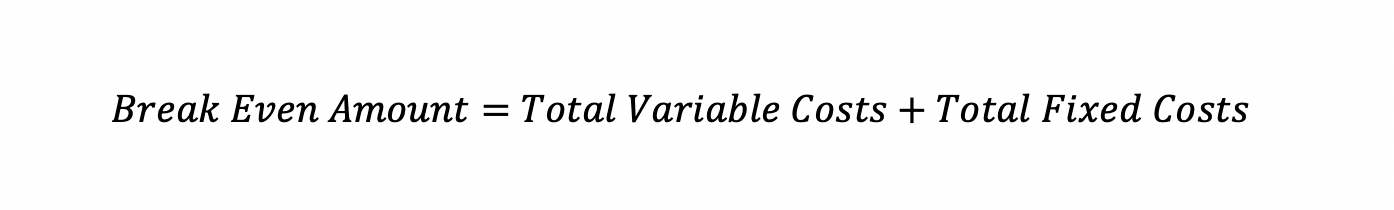To calculate the quantity of the break-even point, we use the following formula:Another way to calculate the break-even point in units is to look at the break-even equation: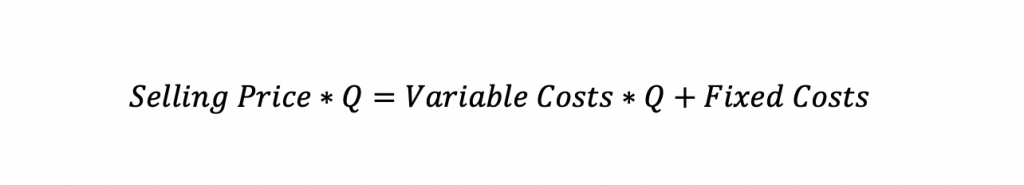By solving the equation for Q, we can find the break-even point in volume of units.

## Targeted income

We can also calculate the CVP equation to get the required sales volume to realize the desired target profit (targeted income).

We add the target income to the Fixed Costs: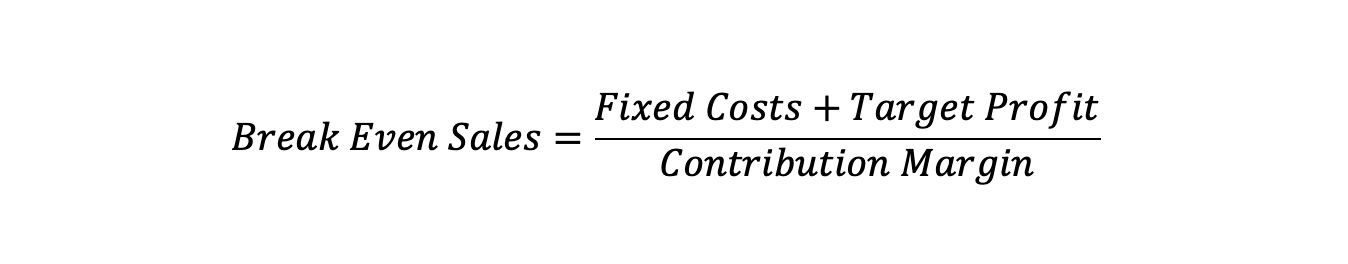If we are calculating the break-even sales for the whole company, and not for a division, we can then also add the income tax we have to cover with sales, to arrive at the targeted income. Then we will adjust the formula as follows: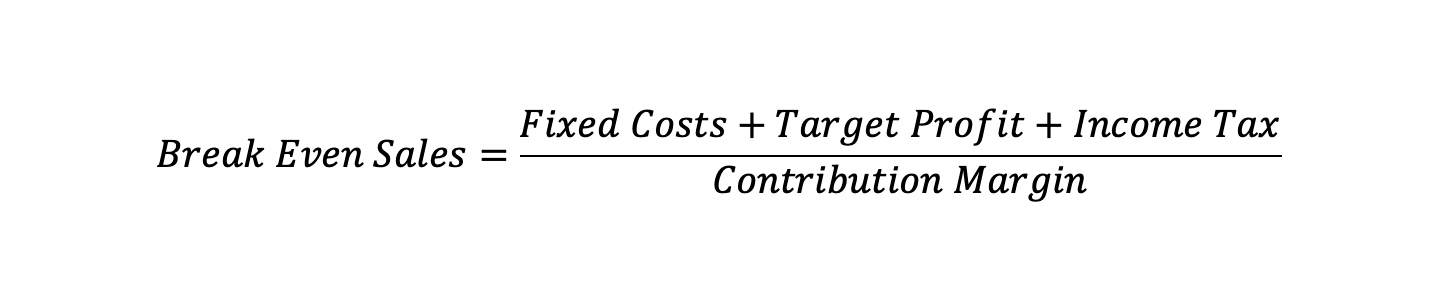# CVP Graph and example

We can also graphically present the CVP analysis. Let us look at an example to create a CVP analysis Graph.

• Total Fixed Costs are 100,000 euros;
• Variable Costs per unit are 2,20 euros;
• Selling Price per unit is 4,00 euros.

Using those we can calculate the Total Costs (Variable and Fixed) and Sales Revenue for different volume levels: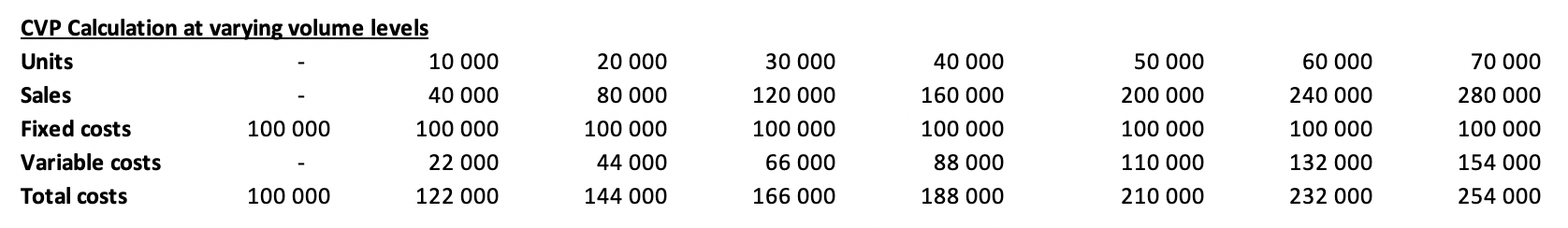We can then calculate the Break-even point using the formulas we discussed above.

Contribution Margin per Unit = Selling Price – Variable Costs per unit
= 4.00 – 2.20
= 1.80 euros per unit

The Break-even volume is = Total FC / CM per Unit
= 100,000 / 1.80
= 55,556 units

The corresponding break-even sales will be the break-even volume multiplied by the selling price of 4.00 euros, or:

55,556 * 4.00 = 222,222 euros.

To plot this in Excel we use an XY Scatter data series on top of our Line series, and we also present it proportionally from the number of XY Scatter data points (look at the Excel file available for download at the end of the article).

We then get the following chart or CVP Graph:The graphical representation of the CVP analysis shows us the following:

• The blue line shows our sales, increasing as the volume increases, multiplied by the selling price of 4.00 euros;
• The brown line shows fixed costs, which are not affected by sales volume and remain constant;
• The grey line shows our variable costs, at 2.20 euro per unit;
• The yellow line shows total costs (fixed costs + variable costs) for the respective sales volume;
• The break-even point is at the sales volume where sales revenue crosses above the total costs line, which means that we start to generate net income from this point on;
• Based on our calculations, we know that the company will break even when 55,556 units are sold for 222,222 euros.

# Contribution margin income statement

Let us look at a more financial representation of the CVP analysis. If we present the calculations in the income statement format, we get the contribution income statement, which is primarily used for internal purposes in companies. By calculating the break-even volume with a targeted pre-tax income of 45,000 euros, we get 80,556 units. Calculating sales and variable costs for this quantity, we get the following:Looking at CVP this way we get a more comprehensive view of required sales volumes to get a 45,000 euros pre-tax income. This income statement shows us that to get the targeted income; we have to achieve the respective sales and keep variable and fixed costs at the specified levels.

# Benefits and Limitations of CVP

The CVP analysis is easy to implement financial analysis technique that can help us with decision making for production volumes. However, we must consider the following benefits and limitations that it faces:

• (+) CVP provides a simple understanding of the sales required to break even or achieve a desired profitability;
• (+) It helps decision-makers with forecasting cost and profit based on activity changes;
• (+) CVP also helps management decide on the optimum levels of production, to maximize profits;
• (-) CVP assumes the scale of production doesn’t affect Total Fixed Costs, which is rarely the case in real-life scenarios;
• (-) Variable Costs are expected to vary proportionally with production/sales volume, which doesn’t happen in reality;
• (-) CVP assumes costs are either Fixed or Variable when in fact a lot of costs are semi-fixed, and their separation to fixed and variable components may not always be achievable.

# Conclusion

The Cost-Volume-Profit analysis is a short-run marginal analysis method that can help us with decision making in regards to optimum production and sales volumes. However, we must keep in mind the assumptions that it makes, which can be hard to set correctly. If the reality deviates too much from the initial assumptions, we might get a CVP analysis that provides us with conclusions that are not very beneficial for the company.

This was a brief view of Cost-Volume-Profit analysis and Break-even point in sales. To review the example calculations, download the attached Excel working file below:## FCCA, FMVA

Hi! I am a finance professional with 10+ years of experience in audit, controlling, reporting, financial analysis and modeling. I am excited to delve deep into specifics of various industries, where I can identify the best solutions for clients I work with.

In my spare time, I am into skiing, hiking and running. I am also active on Instagram and YouTube, where I try different ways to express my creative side.

The information and views set out in this publication are those of the author(s) and do not necessarily reflect the official opinion of Magnimetrics. Neither Magnimetrics nor any person acting on their behalf may be held responsible for the use which may be made of the information contained herein. The information in this article is for educational purposes only and should not be treated as professional advice. Magnimetrics and the author of this publication accept no responsibility for any damages or losses sustained in the result of using the information presented in the publication.